# Quiz 20: The Secondary Mortgage Market: Cmos and Derivative Securities

Business

Duration is the time period in which the annual or monthly payments of a mortgage loan are required to be paid. a. Investment in bond is \$100,000 Compute periodic interest payment as follows:Thus, the periodic interest payment will be \$10,000. Calculate the duration of a corporate bond.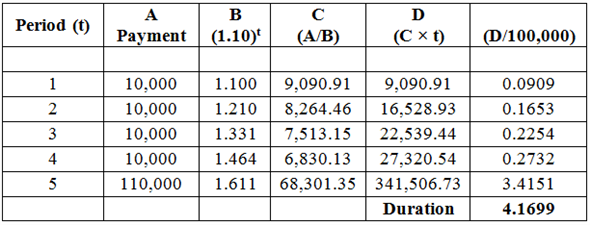Therefore, the duration of the corporate bond is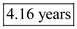. Investment in mortgage bond is \$100,000. Let, assume the annual payment of mortgage bond is \$16,275 and final payment of mortgage bond is \$61,693 in order to attain the actual investment amount of \$100,000.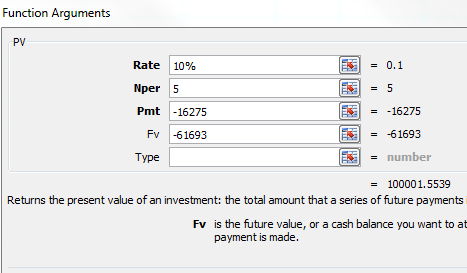Calculate the duration as follows: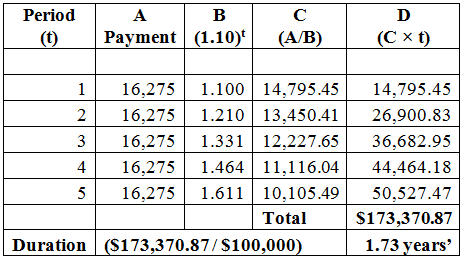Thus, the duration is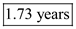. b. Calculate the new price for corporate bond when interest rate falls to 7%.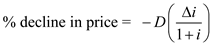Here, The duration is "d". The difference between the old interest and the new interest is "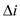". The actual interest rate is "i".Compute percentage decline in price as follows:Thus, the expected decline in price of bond is 11.35%. Compute new price of bond as follows:Therefore, the new price of corporate bond is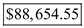. Calculate the new price for mortgage bond when interest rate falls to 7%.Compute percentage decline in price as follows:Thus, the price of bond will decrease by 4.72%. Compute new price of bond as follows:Therefore, the new price of corporate bond is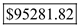.

a. Use spreadsheet for the required computations. Answers are highlighted in 'Orange'. Enter given values and formulas in spreadsheet as shown in the images below. Compute weighted average coupon in the following steps: Firsts step: Compute annual payment for mortgage pool.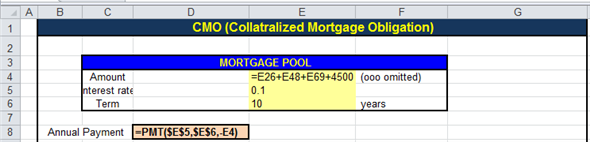Image - A.1 Obtained results are shown below.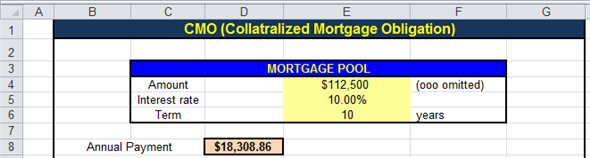Image - A.2 Second step: Prepare loan schedule for mortgage pool.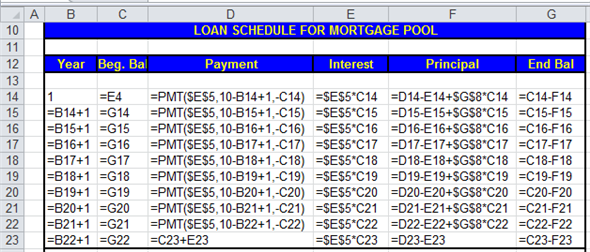Image - A.3 Obtained results are shown below.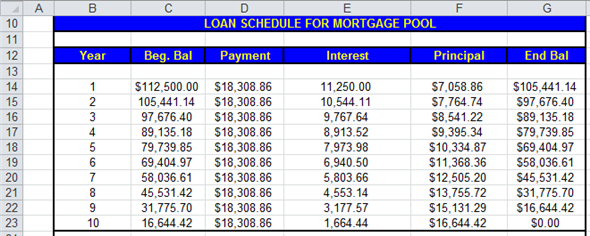Image - A.4 Third step: Compute price of Class Z Tranche.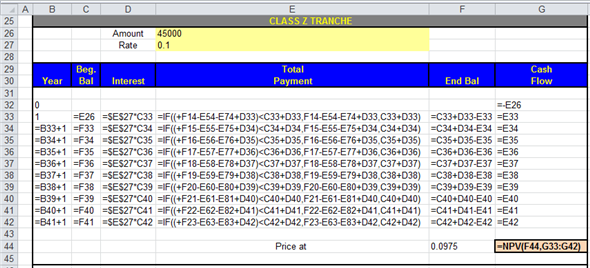Image - A.5 Obtained results are shown below.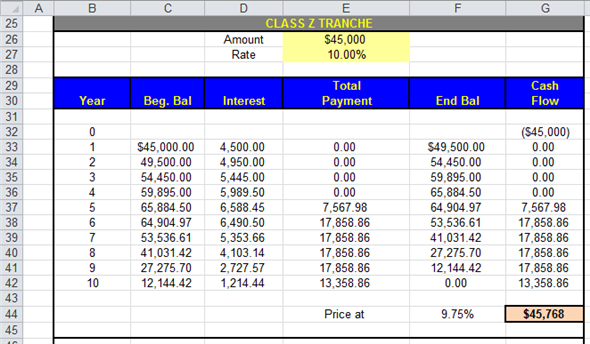Image - A.6 Fourth step: Compute price of Tranche A.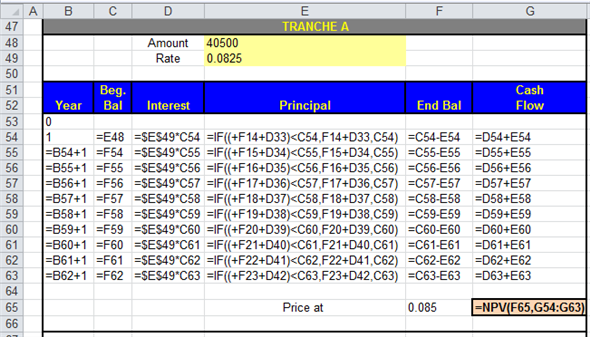Image - A.7 Obtained results are shown below.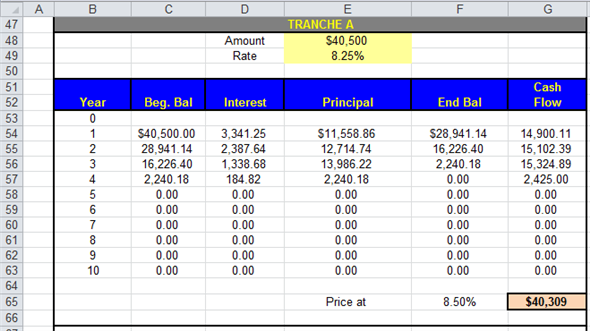Image - A.8 Fifth step: Compute price of Tranche B.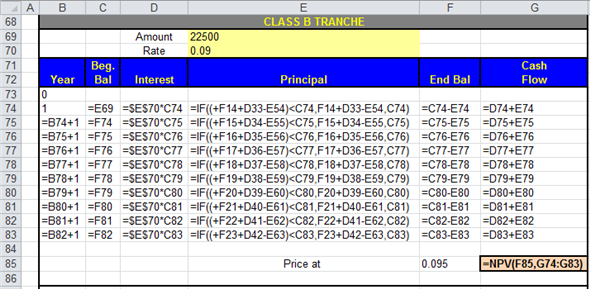Image - A.9 Obtained results are shown below.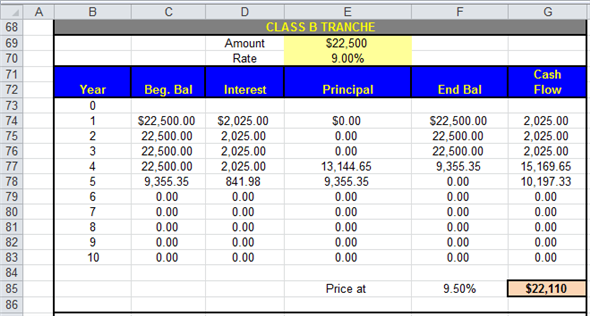Image - A.10 Sixth step: Compute IRR (Internal Rate of Return) for equity over collateralized.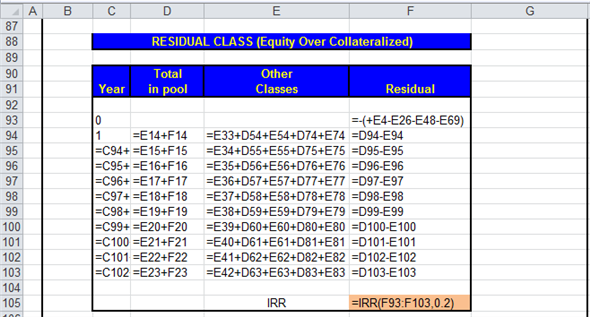Image - A.11 Obtained results are shown below.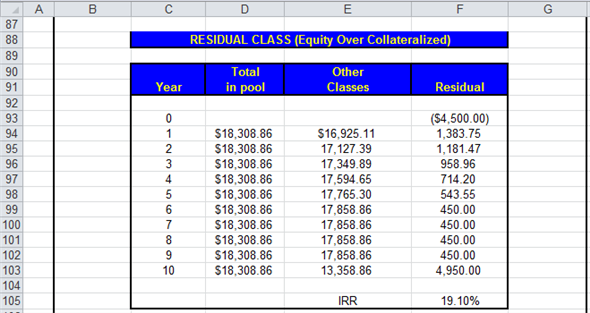Image - A.12 Seventh step: Compute weighted average coupon for all class.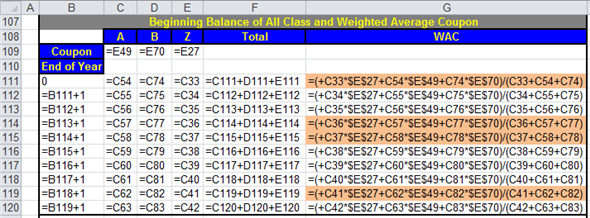Image - A.13 Obtained results are shown below.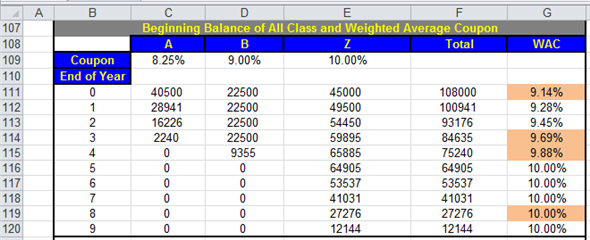Image - A.14 It is evident from the Image - A.14, the weighted average coupon at the time of issue is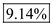. b. It is evident from the Image - A.6, the maturity of Tranche Z is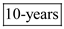. It is evident from the Image - A.8, the maturity of Tranche A is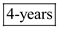. It is evident from the Image - A.10, the maturity of Tranche B is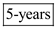. c. It is evident from the Image - A.14, the weighted average coupon at the end of year-3, year-4, and year-8 are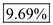,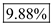, and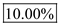respectively. d. It is evident from the Image - A.6, the price of Tranche Z is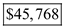. It is evident from the Image - A.8, the price of Tranche A is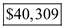. It is evident from the Image - A.10, the price of Tranche B is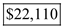. e. The residual cash flow to the issuer is provided in the Image - A.12, and the same is shown below.Image - A.12 Thus, the IRR to the issuer on residual is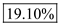. f. Recalculate values for subparts (b) through (e). Firsts step: Compute annual payment for mortgage pool.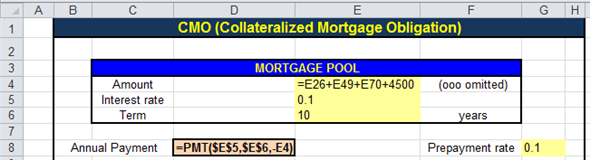Image - A.15 Obtained results are shown below.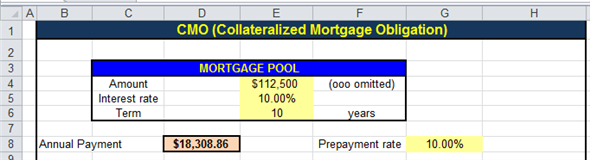Image - A.16 Second step: Prepare loan schedule for mortgage pool.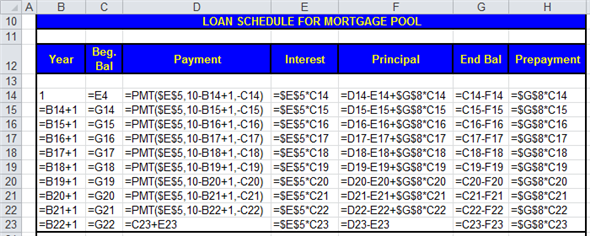Image - A.17 Obtained results are shown below.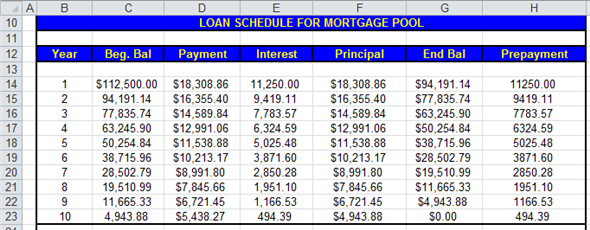Image - A.18 Third step: Compute price of Class Z Tranche.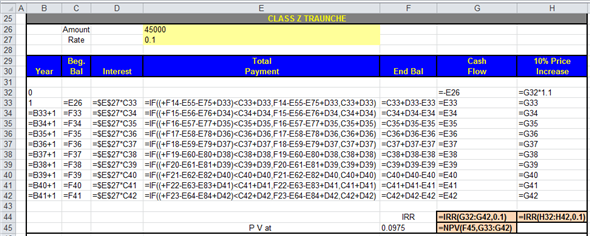Image - A.19 Obtained results are shown below.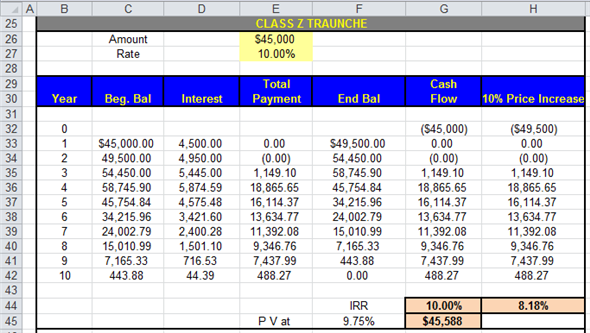Image - A.20 Fourth step: Compute price of Tranche A.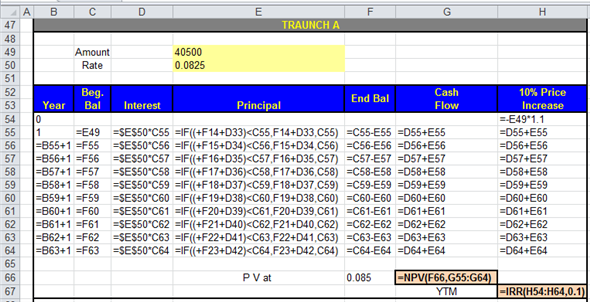Image - A.21 Obtained results are shown below.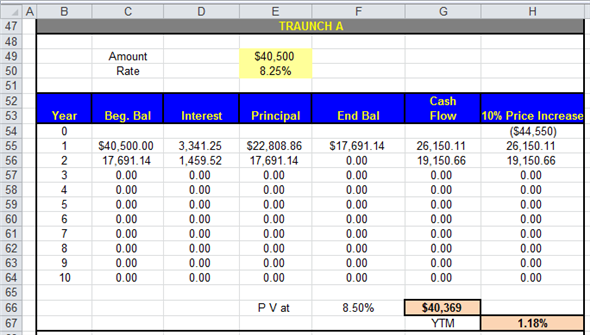Image - A.22 Fifth step: Compute price of Tranche B.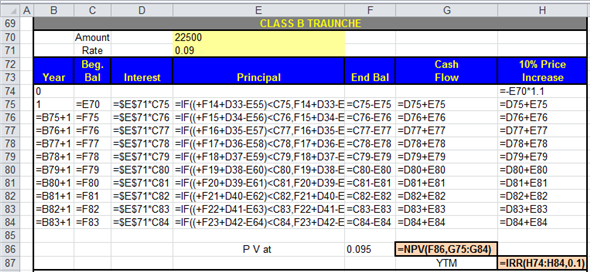Image - A.23 Obtained results are shown below.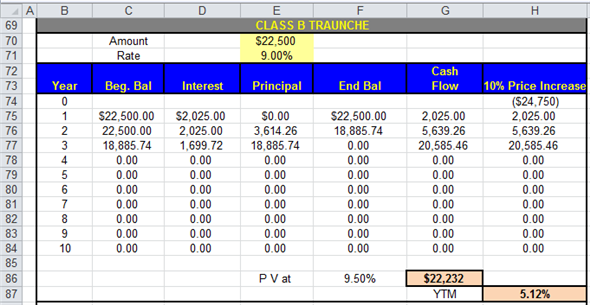Image - A.24 Sixth step: Compute IRR (Internal Rate of Return) for equity over collateralized.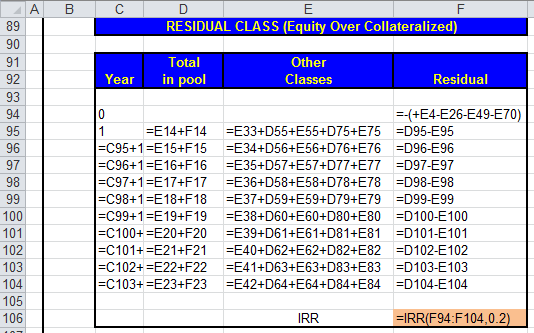Image - A.25 Obtained results are shown below.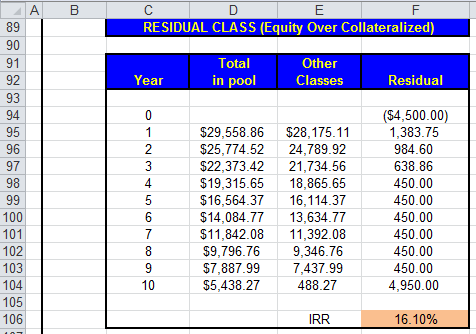Image - A.26 Seventh step: Compute weighted average coupon for all class.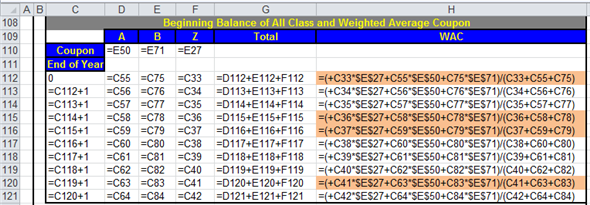Image - A.27 Obtained results are shown below.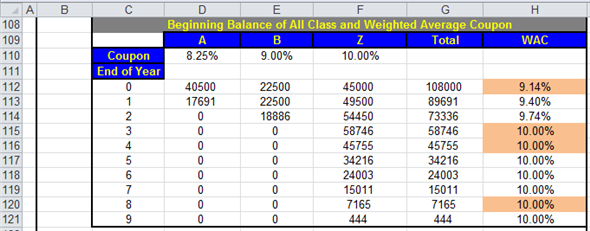Image - A.28 f - b. It is evident from the Image - A.20, the maturity of Tranche Z is. Thus, there is no change for this security. It is evident from the Image - A.22, the maturity of Tranche A is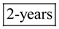. Thus, the maturity of this security has got reduced by. It is evident from the Image - A.24, the maturity of Tranche B is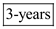. Thus, the maturity of this security has got reduced by. f - c. It is evident from the Image - A.28, the weighted average coupon at the end of year-3, year-4, and year-8 isonly. Thus, for the period of year-3 and year-4 it got increased by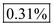and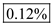respectively. f - d. It is evident from the Image - A.20, the price of Tranche Z is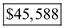. Thus, the price of this security falls by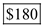. It is evident from the Image - A.22, the price of Tranche A is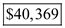. Thus, the price of this security rises by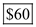. It is evident from the Image - A.24, the price of Tranche B is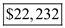. Thus, the price of this security rises by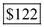. f - e. The residual cash flow to the issuer is provided in the Image - A.26, and the same is shown below.Image - A.26 Thus, the IRR to the issuer on residual is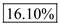. g. It is evident from the Image - A.20, the YTM (Yield to Maturity) of Tranche Z is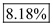. It is evident from the Image - A.22, the YTM (Yield to Maturity) of Tranche A is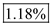. It is evident from the Image - A.24, the YTM (Yield to Maturity) of Tranche B is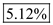.

Mortgage pay through bond security Mortgage pay through bond security is a type of hybrid bond which constitute property of mortgage backed security and mortgage pass through security. This type of security is issued by making the pool of mortgage and the earning and capital gain on mortgage is distributed among the investors in the proportion of investment. Mortgage pay through bond security is a bond security not an equity security. So the creator of this type of security is obliged to pay interest to the investors but the investors have not any ownership like equity holder in the pool of investment. The issuer of Mortgage pay through bond security keeps ownership on the investment with himself. One of the benefits for investors in these type mortgage securities is that the risk level for investors is very less. The level of risk in mortgage backed security is very high compared to Mortgage pay through bond security. Mortgage backed security is issued in term of equity whereas Mortgage pay through bond security is issued in term of bonds.